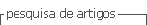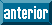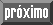## Artigo

•Citado por SciELO
•Acessos

•Similares em SciELO

## versão impressa ISSN 1665-2738

### Rev. Mex. Ing. Quím vol.11 no.2 Ciudad de México Ago. 2012

Simulación y control

Iterative learning estimation of a parameterized input trajectory to control fedbatch fermentation processes: a case study

Estimación por aprendizaje iterativo de una trayectoria de entrada parametrizada para el control de procesos de fermentación en modo fedbatch: un caso de estudio

C. Ben Youssef1* and A. Zepeda2

1Instituto Tecnológico de Cancún, Av. Kabah km 3, C.P. 77500, Cancún, Q. Roo, México.*Corresponding author. E-mail: cherifby@itcancun.edu.mx Tel. +52-998-880-7432x115, Fax +52-998-880-7433

2 Universidad Autónoma de Yucatán. Facultad de Ingeniería Química, Campus de Ingenierías y Ciencias Exactas, periférico norte km 33.5 C.P. 97203, Mérida, Yucatán, México

Accepted May 16, 2012.

Abstract

A novel type of iterative learning control (TLC) strategy for the optimization of fedbatch fermentation processes is developed and applied to lactic acid production as case study. Due to the fact that fedbatch reactors are permanently in transient regime, the tracking behavior of conventional ILC deteriorates as the number of off-line measurements decreases. The first contribution of this study was to use an interpolation method in order to reconstruct the input continuity between the measurement samples. Then, control performance was improved by using an ILC algorithm based on the parameterization of the input profile with piecewise continuous exponential functions that are specially adequate for fedbatch processes. Furthermore, the proposed learning rule was able to track accurately the process output reference with only two off-line measurements. A simulation study demonstrates the feasibility of the ILC approach.

Keywords: iterative learning control, fedbatch, biological process, lactic acid production, simulation.

Resumen

Palabras clave: control por aprendizaje iterativo, fedbatch, proceso biológico, producción de ácido láctico, simulación.

Introduction

Mathematical modeling, identification and real-time control of fermentation processes still represent a challenging urea of endeavor for biotechnologists and control engineers. In particular, the control design is made difficult by at least two well-known major factors. First, the processes involving Living microorganisms exhibit large nonlinearities, strongly coupled variables and often poorly understood dynamics. Second, real-time monitoring of many key process variables, which are needed by advanced control algorithms, is hampered by the lack of reliable on-line sensors (Bastin and Dochain, 1990).

In this work, the attention is focused on fedbatch reactors, in which substrates are fed either intermittently or continuously during the course of fermentation. This operating mode offers many advantages -at least from an industrial viewpoint-over batch and continuous cultures. The main advantage is concretely economical, since improved productivity may be obtained by providing controlled conditions in the supply of inhibitory substrates (Shioya, 1992). However, in contrast to continuous-flow reactors which opérate continuously in steady-state, fedbatch reactors are permanently in a transient regime and therefore present challenging control design problems. Different trends for the control of fedbatch fermentation processes have emerged, principally optimal and adaptive approaches. The main drawback of the first trend is that model-based optimal control method, which provides a theoretically realizable optimum under the assumption of a perfectly known model, could be dangerously misleading in a real-life implementation context. On the other hand, model-independent adaptive controllers do not guarantee a priori optimality of the control policy results. Finally, combining both trends, the approach based on the concept of minimal modeling of the kinetics and that does not suffer from the above difficulties has emerged to fill the gap between modeling accuracy and control needs (Bastin and Dochain, 1990; Van Impe and Bastin, 1995). In these references, it is also demonstrated how optimal -in the sense of optimal productivity- control of fedbatch processes could be replaced by a common nearly optimal regulation control case.

According to this last approach, the control objective is stated in terms of substrate concentration setpoint tracking to fix the influent flow rate and the effective production yield. The output tracking task is then realized by an iterative learning control (ILC) algorithm. The contributions of Arimoto et al. (1984) are widely considered to be the origins of ILC while the main motivation for the development of ILC is the industrial robot (Bien and Xu, 1998; Chen and Wen, 1999). ILC is such a flexible framework that most existing feedback control methods can be used to design the ILC updating Law (Wang et al, 2009), either based on direct or indirect control schemes such as PID-type (Tan et al, 2007), model predictive (Wang et al, 2008a), neural network (Zhang, 2008; Xiong et al, 2010), fuzzy logic (Chien, 2000, 2008), robust (Shi et al, 2006), adaptive (Chien and Tayebi, 2008), fault-tolerant (Wang et al, 2006) or dynamic output feedback (Wang et al, 2008b).

The idea of implementing an ILC strategy to control fedbatch bioprocesses is not new and some interesting results have been reported in the literature, e.g. feedback-assisted Pl-type ILC to control ethanol concentration (Choi et al, 1996), robust ILC to control an exothermic chemical reactor (Mezghani et al, 2001) or ILC based on batch-wise linearized model of a fermentation process (Zhang et al, 2012). The choice of an ILC technique, justified by the repetitive nature of the fedbatch cultures, is motivated by the following advantages upon standard control methods: a) since ILC uses information from previous executions of the task in order to improve the tracking performance from trial to trial, it does not require any on-line measurement, and henee, nor on-line sensor; b) ILC allows limited off-line measurements; c) ILC design is model-independent.

In this work, a lactic acid fermentation process (LAFP) is used as a case study. Lactic acid has traditionally been used in the food industry as an acidulating and/or preservative agent, and in the chemical industry for cosmetic and textile applications (Datta et al, 1995). Fermentation processes, in which lactic acid is obtained from glucose, have currently received much more attention because of the increasing demand for new biomaterials such as biodegradable and biocompatible polylactic producis. The main goal in applying control methods to LAFP systems is to improve operational stability, production efficieney and profit.

A PD-type conventional ILC updating Law is firstly tested, and the simulation study shows how the tracking performances deteriórate as the number of sampling measurements decreases. In order to circumvent this drawback, the same ILC updating Law using piecewise continuous functions (linear and polynomial) to reconstruct the input profile between sampling points is secondly tested (Ben Youssef et al, 2004). Then, an input parameterized ILC controller based on the iterative adjustment of the exponential control profile parameters is designed and implemented. Finally, the convergence property of the algorithm made possible the design of a control strategy that only needs two off-line glucose measurements. Since the main objective of the proposed approach is to improve the feeding profile from run to run, a PD-type ILC controller was chosen rather than a higher order scheme such as PID because the integral action is already operating in the iteration direction. Another reason for this choice is that a PID-type scheme, by increasing the controller order, would increase the complexity of a rigorous convergence proof.

The paper is organized as follows. The general optimal control problem of fedbatch fermentation processes is presented in section 2. The statement of the iterative learning control problem for fedbatch fermentation processes is discussed in section 3. In section 4, the special type of ILC algorithm based on parameterization of the control profile is derived and its performances compared to those of a conventional PD-type ILC scheme. The simulation results are carried out using the case study of a fedbatch lactic acid fermentation process. Concluding remarks end the paper.

2 Fedbatch fermentation optimal control problem

This study considers the class of fedbatch fermentation processes described by a first-order dynamical model (derived from mass balance considerations) of the form:where c, s and p denote respectively the concentrations of cells, limiting substrate and product in the liquid phase of the reactor. φc(·), φs(·) and φp(·) are complex nonlinear functions of the state vector Ξ = [c s p v]' and the constant kinetic parameter vector Θ ∈ Rm. They represent respectively the specific reaction rates of cell growth, substrate consumption and product synthesis. sin is the influent substrate concentration and v is the working volume. The feed flow rate u serves as the input variable of the system.

Fedbatch control problem is an optimal control problem. Since the objective of product synthesis viafermentation processes is to maximize, in a minimal time, the final quantity of product, a global criterion may be given by:where γ1, γ2 and γ3 are weighting coefficients, sƒ v1 is the residual quantity of substrate and Tƒ is the shutdown time. This non-quadratic criterion and additional constraints in the process variables forcé to use techniques that involve iterations towards the optimum, e.g. procedures based on Pontryagin's múximum principie, Green's theorem or dynamical programming. These techniques are not well adapted to implement closed-loop control algorithms, but they allow to determine open-loop optimal profiles of the process variables. However, optimal control of biological systems is a very model-sensitive approach. For instance, besides a perfect analytical expression of the specific reactions rates, i.e. φc(·), φs(·) and φp(·), an optimal control algorithm would require on-line measurement of the full state vector ≡ In biotechnological applications, these assumptions are in practice never fulfilled.

A more realistic and practical solution consists in implementing closed-loop controllers which regulate one -if possible- process variable. The aim of such sub-optimal strategy is to replace the given optimal problem by a more common regulation one. Then, this standard control problem can be solved by most of conventional feedback control loops, e.g. a PI control of fedbatch phenol degradation in Leonard et al. (1999) or adaptive linearizing control of fedbatch Penicillin G production in Van Impe and Bastin (1995).

3 Fedbatch fermentation control with ILC

In most industrial fedbatch fermentation applications, the operating cycle is executed in a finite time interval while the same operation will be repeated (see Fig. 1). It should also be pointed out that industrial fedbatch production is traditionally carried out in open-loop conditions using precalculated substrate feeding profiles. In such a case, the idea of iterative learning control is intuitively clearly applicable to improve the fedbatch reactor performances from run to run. Furthermore, when only partial knowledge about the plant is available, the learning feature of the ILC approaches serves as a bridge between knowledge and experience. Moreover, as mentioned in the introduction, classical ILC approach does not require on-line measurements for its implementation, circumventing thus the insufficient measurement capability in most industrial fermentation systems, and fedbatch LAFP in particular. Although ILC only modifiles the input command for the next operation, it should be pointed out that it is actually not an open-loop control operation (Arimoto et al, 1984). While conventional controllers are closed-loop in time, ILC is closed-loop in iterations since updates are performed for the next iteration using the measurements of the previous iteration.Let an operation of the fedbatch system to be controlled be denoted by subscript i and let the time during a given trial be denoted by t, where t ∈ [0,Tƒ]. The usual way to implement ILC is to use the following general updating formula for the input signal Ui(t):where H(q) and L(q) are linear filters, not necessary causal, and q is the time-delay operator. ei (t)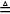yd (t) - yi (t) is the output tracking error and yd(t) the desired output trajectory.

Let tk = {kT}k=1,...,N denotes the discrete interval, N being the number of off-line measurements and T the sampling period. The ILC's objective is to derive an optimal input sequence ud(t) for t ∈by evaluating the error I to adjust the input ui(tk) for the next trial, such that yi (t)yd(t) when i∞. ui+1+i(i) for t ∈ [tk tk+1]is usually generated by a zero-order hold (ZOH) function.

According to the discussion presented in section 2, the initial optimal fedbatch control problem, which corresponds to the maximization of the performance criterion given by Eq. (2), is replaced by a scalar output regulation problem at some sub-optimal setpoint y*. In order to satisfy the standard ILC reset conditions, i.e. yi (0) = yd (0) = y0 for all i, the desired output trajectory is generated by the following first-order filter:The choice of a low substrate concentration control strategy is common in fermentation applications since it avoids nutrient spilling and cell growth limitation due to product accumulation ( Van Impe and Bastin, 1995; Leonard et al, 1999; Ben Youssef et al, 2000). In this study, the glucose setpoint value is set to y* = 3 gL-1, and the desired output trajectory is generated by using Eq. (4) with λ = 2.

4 Case study: the fedbatch LAFP

4.1 The LAFP model

The dynamical model of the fedbatch lactic acid fermentation process used in this paper is of the form of Eq. (1). The process consists in lactic acid (p) production by Lactobacillus casei (c) on glucose (s). More details about the LAFP process are given in Olmos-Dichara et al. (1997). The specific reaction rates are modelled as follows: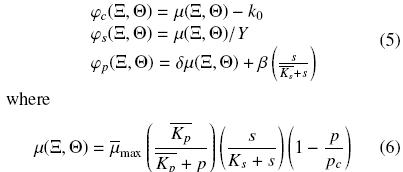The dependency of the parameters according to the so-called growth factor a -denoted by an overbar- is described by: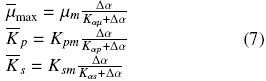where Δα = α - α0. The model was designed by Ben Youssef et al. (2005) and validated for six experimental batch cultures corresponding to different values of growth factor a, which may be considered as a secondary substrate. The case α = 2 is arbitrary chosen for fedbatch control simulations which are obtained by integrating Eq. (1) using eqs. (5)-(7). The meanings and values of the biological variables and parameters are given in Table 1. Initial conditions are x(0) = 0.2 gL-1, s(0) = 50 gL-1, p(0) = 0 gL-1, a(0) = 2 and v(0) = 2 L. All the simulation runsare carried out assuming in the first iteration the worst case of a priori knowledge about the optimal input trajectory ud(t) by setting u0(t) = 0 for all t, i.e. by beginning the ILC procedure with a batch operating mode.4.2 Conventional ILC

A conventional ILC based using a ZOH function is firstly applied to glucose concentration control of fedbatch lactic acid fermentation process. The iterative learning adjustment of ui(t) is done by using the discrete PD-type updating formula:where kp and kd are constant learning gains. Fig. 2 illustrates the time evolution at iteration i (for i = 1,..., 15) of the main LAFP variables, i.e the feed flow rate ui(t), the glucose concentration si(t), the lactic acid concentration pi(t), the biomass concentration ci(t) and the control error ei(t). Samples are assumed to be taken each hour (T = 1 h), so the number of glucose measurements is N = 200. In order to visualize the algorithm convergence, the evolution of the 2-norm of the error ||e;(t + Δt)||2 versus iterations i with a time increment Δt = 0.1 h is shown in the same figure.

The learning gains are kp = 0.04 and kd = 0.01. It is shown that convergence of the output to the desired trajectory yd(t) is obtained after approximately 12-13 iterations. No a priori knowledge about the process model was necessary for the ILC algorithm to control satisfactorily the fedbatch LAFP system. ||ei;(t)||2 does not converge to zero because of the accumulation of errors during the initial lag phase of substrate consumption, i.e. t ∈ [0,t1]where t1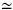30 h. During this initial time interval, the input is saturated at ui(t) = 0 for all i, and because the glucose consumption dynamic is slower than that of the desired trajectory yd(t), it is not possible to reduce this error.

It has already been demonstrated that a substrate or product regulation policy at a given optimal setpoint yields to an exponential evolution of the corresponding optimal feed flow rate (Lee et al, 1999; Leonard et al, 1999). This is confirmed by the exponential profile of u(t) as shown in Fig. 2. It is important to point out that the exact exponential function of the process input is a priori unknown and that it took about 15 iterations for the conventional ILC scheme to achieve convergence. However, for practical and economical purposes, the sampling period of T= 1 h is not acceptable, since it corresponds to an excessive number of off-line glucose laboratory analysis, i.e. N = 200 for each single operation i. Henee, the fact of increasing period T is imposed by the important economic aspect of minimizing N -and consequently, the operator availability- in a real-life implementation.

The same ILC algorithm based on ZOH function is used in a new simulation (see Fig. 3) with an increased sampling period T = 25 h and a corresponding reduced number of off-line analysis, i.e. N = 8. As shown in this figure, the conventional ILC procedure has not produced acceptable control performances since the inherent zero-order hold input policy does not allow continuity in the input profile, and consequently nor in the output profile. This undesired behavior is reflected by the convergence of the error ||ei(t)||2 to a bigger value than that obtained in the case of N = 200. This ocurrence can easily be explained by the fact that the substrate control goal for fedbatch fermentation processes consists in keeping an inherently unstable type of behavior under control (Bastin and Dochain, 1990; Van Impe and Bastin, 1995), which in turn results in time-varying profile of the actual process variables. Henee, freezing the control input during a too large period of time comes against its natural time-varying behavior and could lead, as shown in these simulation results, to misleading control trajectories.

4.3 Input interpolation

In this context, the idea of using some interpolation method in order to reconstruct a continuous input profile between sampling points arises to circumvent the convergence problems due to the zero-order hold input policy in conventional ILC updating Laws. In Fig. 3, simulation results are illustrated for linear and cubic-polynomial piecewise continuous function for input interpolation.

Output convergence to the desired glucose reference model is observed after approximately 7-8 iterations. Improved transient behavior and convergence rate are obtained in both cases, while the difference between the two algorithm performances is not significant. However, the final value of ||ei(t)||2 is still bigger than the value obtained in the case of conventional ILC algorithm with N = 200 (see Fig. 2). The increased error is generated by the small overshoot observed a t35 h in Fig. 3, which in turn is due to the time localization mismatch between the first sampling period t = 25 h and the time the feeding pump is supposed to begin to actuate, i.e. t130 h h. Although this kind of behavior may be avoided by adjusting the measurement period to an estimated value of t1, this solution comes against the assumption of no a priori process knowledge context of this study. Furthermore, since the initial lag phase t1 is directly correlated to the initial concentrations of the main LAFP process variables, its pre-estimation would be necessary each time the operating initial conditions are changed.

4.4 ILC with input parameterization

Following the idea of dynamic fitting (Chen et al, 1997; Xu et al, 1999), instead of solving a functional minimization problem according to conventional ILC approach, one may parameterize the control ui(t) as: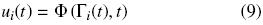where Φ(·) ∈ R a properly chosen basis function and Γi(t)Rp an input parameter vector. The remaining task consists then in estimating the control parameters through an iterative learning Law. At this stage, and considering the specific case of fedbatch fermentation processes, the main difficulty remains in an appropriate choice of the parameterized input function Φ(·). Taking into account the previously mentioned fact that a substrate regulation strategy at the optimal setpoint y* in fedbatch mode results in an a priori unknown exponential evolution of the feed flow rate, let the input function ui(t) have the following delayed piecewise continuous exponential form:where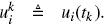. The input parameter vectoris updated at each iteration i through the following first-order iterative learning law:where L1 is the learning gain vector to be designed. In order to be able to compare the efficiency of this new ILC control law and that of the conventional scheme of Eq. (8), the same LAFP initial conditions and the case N = 8 are assumed.

The parameterized ILC control algorithm, with L1 = [-0.5,0.01,0.015I7]' where I7 is the 1 x 7 unit vector, demonstrates a perfect tracking after 6-7 iterations as shown in Fig. 4. It can also be observed that the input parameters converge to their corresponding final optimal value with the same rate.

It is important to notice that, when compared to the results of the conventional PD-type ILC scheme, besides the improvement of the iterative learning convergence rate, the transient behavior of the output tracking has also been improved. One of the important feature of the control law given by Eq. (10) is that the error due to an inaccurate selection of the sampling period (as illustrated in the previous example) is eliminated by considering the first sampling time t1 as an additional parameter to be iteratively estimated. Finally, the algorithm still exhibits the important feature of ILC approaches, which is the possibility of using a reduced number of off-line measurements, i.e. only 8 samples for a 200 hours LAFP operating cycle. It is interesting to note how all theparameters in Fig. 4 converge to the same constant optimal value a*0.01. This convergence characteristic confirms that the learning process of the ILC algorithm was correct. Taking this occurrence under consideration, the following continuous exponential function for the parameterization of the ILC control law is proposed:where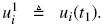. Only three input parameters are needed to be estimated via iterative learning, i.e.. Consequently, since the choice of a control law of the form of Eq. (12) implies that N = 2, only two off-line measurements are needed for its implementation. It is shown in Fig. 5 that the parameterized ILC control law, with L1 = [-0.5,0.01,0.015]' , is able to achieve acceptable convergence rate. For instance, the subfigures corresponding to the evolution of the input parameters versus the number of iterations illustrate how the corresponding initial values are close to zero and how they converge quite rapidly, i.e. after only about 5-6 iterations, to their unknown optimal values. Although convergence rate has been improved when compared to the results of the conventional PD-type ILC, the main advantage of this approach is that the number of off-line measurements has been drastically reduced. This characteristic is obviously of great importance from the practical and economical point of view when controlling fedbatch fermentation processes. It should be finally mentioned that cell growth control by using an exponential feeding pattern has allowed productivity and yield to be increased in several other biotechnological processes (Ho et al, 1999; d'Anjou and Daugulis, 2000).

Remark. The boundedness of trajectories during each iteration is guaranteed theoretically because only a finite time interval is concerned in every iteration (Chen and Wen, 1999). However, in practice, it may happen that the tracking errors in between sampling points are large while maintaining a good pointwise tracking, as illustrated in Fig. 2 and Fig. 3. In the case of Eq. (12), the boundedness of the error trajectories with only 2 sampling points is still guaranteed because the exponential input profile is sufficiently close to that of the optimal one.

To demonstrate practical robustness of the proposed scheme, a simulation with non-repetitive parametric disturbances m is set to 0.55 after 9 iterations) and 10% additive zero-mean signal noise for more realistic measurement is run as shown in Fig. 6. Significant robustness of the ILC controller is demonstrated by its efficiency of rejecting the signal noise effects and its capacity of absorbing the parametric perturbation after only 3-4 iterations.

These case study results show the applicability of the proposed approach. A rigorous convergence proof of the algorithm should be carried on by combining the convergence results based on dynamical fitting (Chen et al, 1997; Xu et al, 1999) together with those of Chien (2000) for ILC of nonlinear sampled systems.

5 Conclusions

Based on the idea of dynamic fitting, a novel type of ILC strategy using an appropriate exponential parameterization of the input profile, is designed and compared to a conventional ILC PD-type one. The simulation results, using the case study of a fedbatch lactic acid fermentation process without loss of generality, show that improved ILC convergence rate and transient behavior are obtained. Furthermore, only two off-line measurements are necessary for its implementation, showing the practical and economical advantage of this ILC strategy upon standard ones.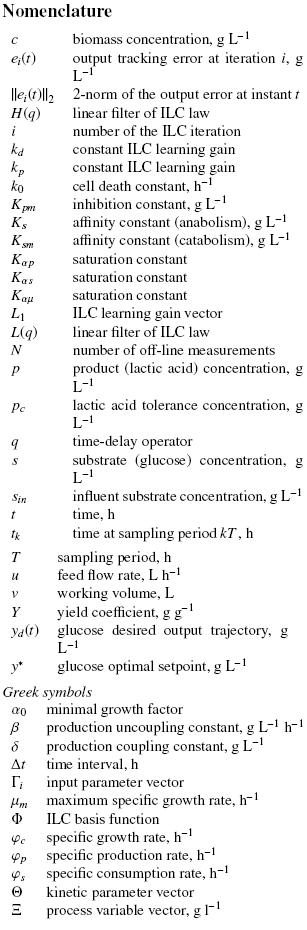References

Arimoto, S., Kawamura, S. and Miyazaki, F. (1984). Bettering operation of robots by learning. Journal of Robotic Systems 1, 123-140.         [ Links ]

Bastin, G. and Dochain, D. (1990). On-line Estimation and Adaptive Control of Biore actors. Elsevier, Amsterdam.         [ Links ]

Ben Youssef, C, Guillou, V. and Olmos-Dichara, A. (2000). Modelling and adaptive control in a lactic fermentation process. Control Engineering Practice 8, 1297-1307.         [ Links ]

Ben Youssef, C, Goma, G. and Olmos-Dichara, A. (2005). Kinetic modelling of Lactobacillus casei ssp. rhamnosus growth and lactic acid production in batch cultures under various médium conditions. Biotechnology Letters 27, 1785-1789.         [ Links ]

Ben Youssef, C, Waissman, J., VÃ¡zquez, G. and López V. (2004). Iterative learning control with input parameterization of a fedbatch lactic acid fermentation process. WSEAS Conference on Mathematics 3, 49-54. April 21-23, Miami, Florida.         [ Links ]

Bien, Z. and Xu, J. X. (1998). Iterative Learning Control: Analysis, Design, Integration and Applications, Kluwer Academic Publishers, Boston.         [ Links ]

Chen, Y. and Wen, C. (1999). Iterative Leaming Control: Convergence, Robustness and Applications, Springer-Verlag, Heidelberg.         [ Links ]

Chen, Y., Wen, C, Gong, Z. and Sun, M. (1997). Drag coefficient curve identification of projectiles from flight tests via optimal dynamic fitting. Control Engineering Practice 5, 627-636.         [ Links ]

Chien, C.-J. (2008). A combined adaptive law for fuzzy iterative leaming control of nonlinear systems with varying control tasks. IEEE Transactions on Fuzzy Systems 16, 40-51.         [ Links ]

Chien, C.-J. (2000). A sampled-data iterative leaming control using fuzzy network design. International Journal of Control 73, 902-913.         [ Links ]

Chien, C.-J. and Tayebi, A. (2008). Further results on adaptive iterative leaming control of robot manipulators. Automatica 44, 830-837.         [ Links ]

Choi, J.-W., Choi, H.-G., Lee, K.-S. and Lee, W-H. (1996). Control of ethanol concentration in a fed-batch cultivation of Acinetobacter calcoaceticus RAG-1 using a feedback-assisted iterative leaming algorithm. Journal of Biotechnology 49, 29-43.         [ Links ]

d'Anjou, M. C. and Daugulis, A. J. (2000). Mixed-feed exponential feeding for fed-batch culture of recombinant methylotrophic yeast. Biotechnology Letters 22, 341-346.         [ Links ]

Datta, R., Tsai, S. R, Bonsignore, R, Moon, S. H. and Frank, R. J. (1995). Technological and economic potential of poly (lactic acid) and lactic acid derivatives. FEMS Microbiology Reviews 76,221-231.         [ Links ]

Ho, K. R, Tam, C. and Zhou, B. (1999). Growth and carotenoid production of Phaffia rhodozyma in fed-batch cultures with different feeding methods. Biotechnology Letters 21, 175-178.         [ Links ]

Lee, J., Lee, S. Y, Park, S. and Middelberg, A. R J. (1999). Control of fed-batch fermentations. Biotechnology Advances 17, 29-48.         [ Links ]

Leonard, D., Ben Youssef, C, Destruhaut, C, Lingley, N. D. and Queinnec, I. (1999). Phenol degradationby, Ratooraa eutropha: colorimetric determination of 2-hydroxymuconate semialdehyde accumulation to control feed strategy in fed-batch fermentations. Biotechnology and Bioengineering 65, 407-415.         [ Links ]

Mezghani, M., Roux, G., Cabassud, M., Dahhou, B., Le Lann, M. V. and Casamatta, G. (2001). Robust iterative leaming control of an exothermic semi-batch chemical reactor. Mathematics and Computers in Simulation 57, 367-385.         [ Links ]

Olmos-Dichara, A., Ampe, F., Uribelarrea, J. L., Pareilleux, A. and Goma, G. (1997). Growth and lactic acid production by Lactobacillus casei ssp Rhamnosus in batch and membrane bioreactor. Biotechnology Letters 19, 709-714.         [ Links ]

Shi, J., Gao, F. and Wu, T.-J. (2006). Robust iterative learning control design for batch processes with uncertain perturbations and initialization. AIChE Journal 52, 2171-2187.         [ Links ]

Shioya, S. (1992). Optimization and control in fed-batch bioreactors. Advances in Biochemical Engineering Biotechnology 46, 111 -142.         [ Links ]

Tan, K. K., Zhao, S. and Xu, J.-X. (2007). Online automatic tuning of a proportional integral derivative controller based on an iterative leaming control approach. IET Control Theory Applied 1,90-96.         [ Links ]

Van Impe, J. F. and Bastin, G. (1995). Optimal adaptive control of fed-batch fermentation processes. Control Engineering Practice 3, 939-954.         [ Links ]

Wang, Y, Gao, F. and Doyle, F. J. (2009). Survey on iterative learning control, repetitive control, and run-to-run control. Journal of Process Control 19, 1589-1600.         [ Links ]

Wang, Y. Q., Shi, J., Zhou, D. H. and Gao, F. R. (2006). Iterative learning fault-tolerant control for batch processes. Industrial and Engineering Chemistry Research 45, 9050-9060.         [ Links ]

Wang, Y. Q., Zhou, D. H. and Gao, F. R. (2008a). Iterative learning model predictive control for multi-phase batch processes. Journal of Process Control 18, 543-557.         [ Links ]

Wang, Y. Q., Zhou, D. H. and Gao, F. R. (2008b). Iterative learning reliable control of batch processes with sensor faults. Chemical Engineering Science 63, 1039-1051.         [ Links ]

Xiong, Z., Xu, Y., Dong, J. and Zhang, J. (2010). Neural network based iterative learning control for product qualities in batch processes. International Journal of Modelling, Identification and Control 11, 107-114.         [ Links ]

Xu, J.-X., Chen, Y., Lee, T. and Yamamoto, S. (1999). Terminal iterative control with an application to RTPCVD thickness control. Automatica 35, 1535-1542.         [ Links ]

Zhang, J. (2008). Batch-to-batch optimal control of a batch polymerisation process based on stacked neural network models. Chemical Engineering Science 63, 1273-1281.         [ Links ]

Zhang, J., Xiong, Z., Delautre, G. and Lamande, A. (2012). Batch to batch iterative leaming control of a fed-batch fermentation process. Mechanical Engineering and Technology 125, 253-260.         [ Links ]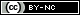Todo o conteúdo deste periódico, exceto onde está identificado, está licenciado sob uma Licença Creative Commons